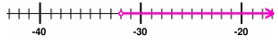# Graphing a Linear Inequality on the Number Line

We represent inequalities by using a number line in this lesson.

• In the examples below, we show the range of true values for a given inequality.

• We use an open dot to represent < and > relationships; this symbol indicates that the point on the number line is not included within the range of possible values for the inequality.

• We use a closed dot to represent ≤ and ≥, when the two sides of the inequality could be equal.

Number Line

We recall that a number line is a horizontal line that has points, which correspond to numbers. The points are spaced according to the value of the number they correspond to; the points are equally spaced in a number line containing only whole numbers or integers.

Graph of the point “3”

We graph numbers by representing them as points on the number line. For example, we graph "3" on the number line as shown below −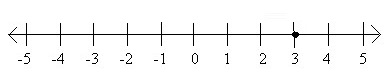Graph of the Inequality x ≤ 3

We can also graph inequalities on the number line. The following graph represents the inequality x ≤ 3. The dark line represents all the numbers that satisfy x ≤ 3. If we pick any number on the dark line and plug it in for x, the inequality will be true.Graph of the Inequality x < 3

The following graph represents the inequality x < 3. Note that the open circle on 3 shows that 3 is not a solution to x < 3.Graph of the Inequality x > 3

Here are the graphs of x > 3 and x ≥ 3, respectively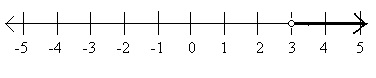Graph of the Inequality x ≥ 3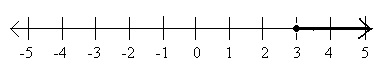Graph of the Inequality x ≠ 3

An inequality with a “≠" sign has a solution set which is all the real numbers except a single point. Thus, to graph an inequality with a " ≠ " sign, graph the entire line with one point removed. For example, the graph of x ≠ 3 looks like −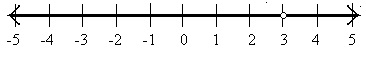Graph the following inequality on the number line −

$\frac{x}{3}$ ≤ 7

### Solution

Step 1:

$\frac{x}{3}$ ≤ 7; x ≤ (7 × 3); x ≤ 21

We first locate the point 21 on the number line.

Step 2:

We put a closed circle on 21 and draw a thick line towards left to denote the inequality x ≤ 21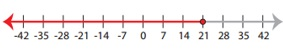Graph the following inequality on the number line −

x > −32

### Solution

Step 1:

We first locate the point −32 on the number line.

Step 2:

We put a open circle on −32 and draw a thick line towards right to denote the inequality

x > −32